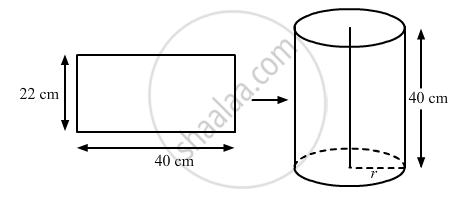# A rectangular sheet of paper 40 cm × 22 cm, is rolled to form a hollow cylinder of height 40 cm. The radius of the cylinder (in cm) is ______. - Mathematics

MCQ
Fill in the Blanks

A rectangular sheet of paper 40 cm × 22 cm, is rolled to form a hollow cylinder of height 40 cm. The radius of the cylinder (in cm) is ______.

#### Options

•  3.5

• 7

• $\frac{80}{7}$

• 5

#### Solution

A rectangular sheet of paper 40 cm × 22 cm, is rolled to form a hollow cylinder of height 40 cm. The radius of the cylinder (in cm) is 3.5.

Explanation:We have,

Length of the rectangular sheet, l = 40 cm,

Width of the rectangular sheet, b = 22 cm and

Height of the hollow cylinder, h = 40 cm

Let the radius of the cylinder be r

As, l = h

So, the circumference of base of the cylinder = b

⇒ 2πr = 22

=> 2xx22/7xx"r" = 22

= "r"=(22xx7)/(2xx22)

∴ r = 3.5 cm

Is there an error in this question or solution?
Chapter 14: Surface Areas and Volumes - Exercise 14.5 [Page 91]

#### APPEARS IN

RD Sharma Class 10 Maths
Chapter 14 Surface Areas and Volumes
Exercise 14.5 | Q 43 | Page 91
RS Aggarwal Secondary School Class 10 Maths
Chapter 19 Volume and Surface Area of Solids
Multiple Choice Questions | Q 14 | Page 919
Share